# How to hide legend with Plotly Express and Plotly in Python?

• Last Updated : 19 Dec, 2021

In this article, we will learn How to hide legend with Plotly Express and Plotly. Here we will discuss two different methods for hiding legend in plotly and plotly express, using two different examples for each to make it more clear.

Syntax: For legend:

• fig.update_traces(showlegend=False)
• fig.update(layout_showlegend=False)

Example 1:

In this example, we are hiding legend in Plotly Express with the help of method fig.update_traces(showlegend=False), bypassing the show legend parameter as False.

## Python3

 `# importing packages ` `import` `plotly.express as px ` ` `  `# using the gapminder dataset ` `df ``=` `px.data.tips() ` `fig ``=` `px.scatter(df, x``=``"total_bill"``, y``=``"tip"``, color``=``"sex"``,  ` `                 ``symbol``=``"smoker"``, facet_col``=``"time"``, ` `                 ``labels``=``{``"sex"``: ``"Gender"``, ``"smoker"``: ``"Smokes"``}) ` ` `  `# hiding legend in pyplot express. ` `fig.update_traces(showlegend``=``False``) ` ` `  `fig.show()`

Output: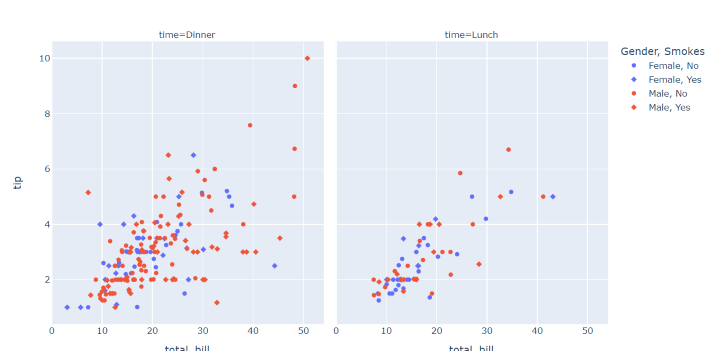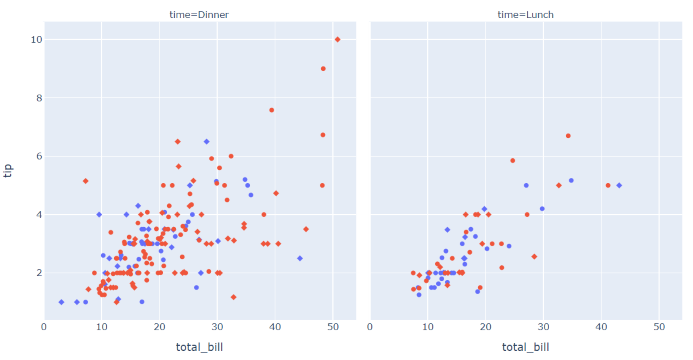Example 2:

In this example, we are hiding legend in Plotly with the help of method fig.update(layout_showlegend=False), by passing the showlegend parameter as False.

## Python3

 `import` `plotly.graph_objects as go ` ` `  `# using the Figure dataset ` `fig ``=` `go.Figure() ` ` `  `fig.add_trace(go.Line(name``=``"first"``, x``=``[``"a"``, ``"b"``], y``=``[``1``,``3``])) ` `fig.add_trace(go.Line(name``=``"second"``, x``=``[``"a"``, ``"b"``], y``=``[``2``,``1``])) ` `fig.add_trace(go.Line(name``=``"third"``, x``=``[``"a"``, ``"b"``], y``=``[``1``,``2``])) ` `fig.add_trace(go.Line(name``=``"fourth"``, x``=``[``"a"``, ``"b"``], y``=``[``2``,``3``])) ` ` `  ` `  `# hiding legend in pyplot express. ` `fig.update(layout_showlegend``=``False``) ` ` `  `fig.show()`

Output: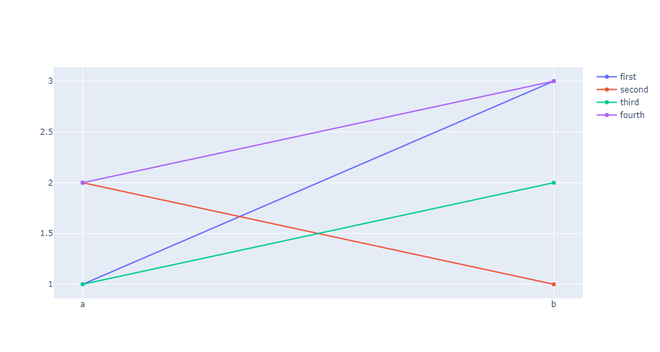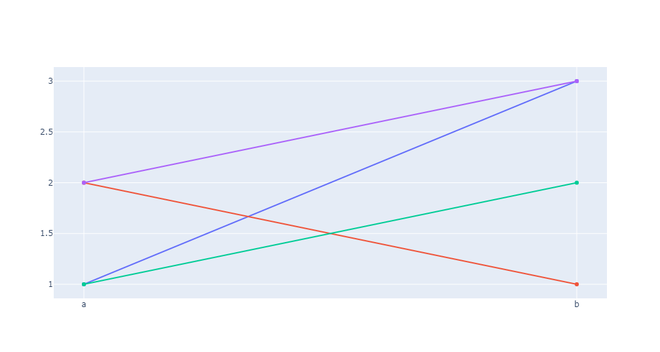Example 3:

In this example, we are hiding legend in Plotly Express with the help of method fig.update_traces(showlegend=False), by passing the layout_showlegend parameter as False.

## Python3

 `import` `plotly.express as px ` ` `  `# using the iris dataset ` `df ``=` `px.data.iris() ` ` `  `# plotting the line chart ` `fig ``=` `px.line(df, y``=``"sepal_width"``, line_dash``=``'species'``, ` `            ``color``=``'species'``) ` ` `  `# hiding legend in pyplot express. ` `fig.update_traces(showlegend``=``False``) ` ` `  `# showing the plot ` `fig.show() `

Output: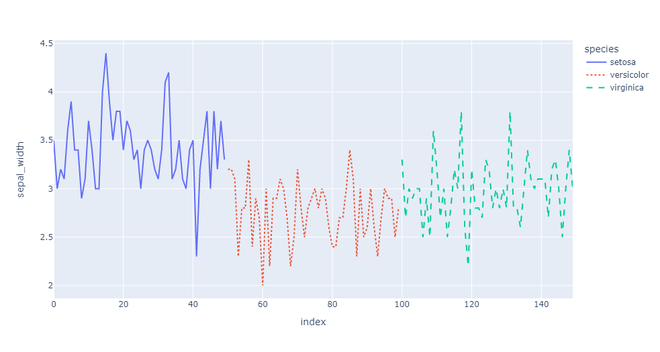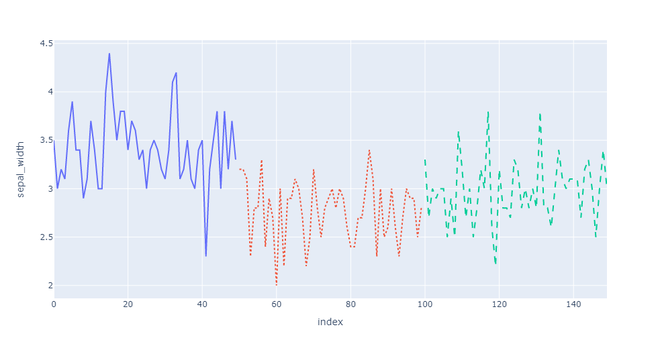My Personal Notes arrow_drop_up
Recommended Articles
Page :